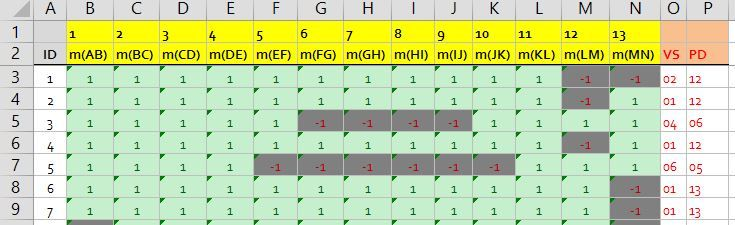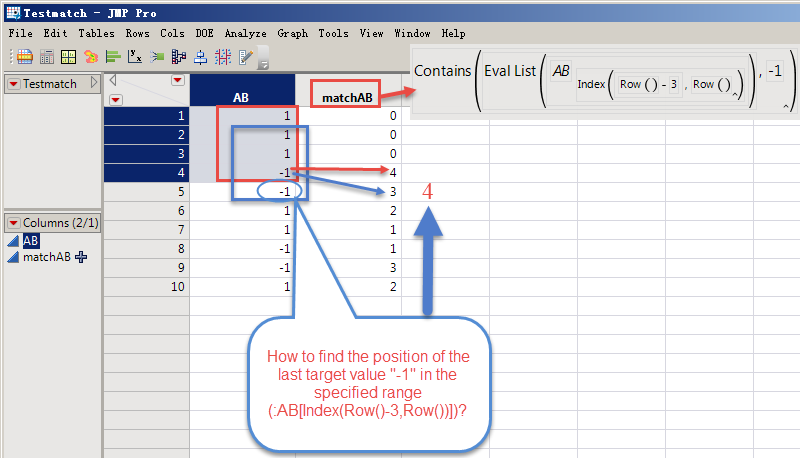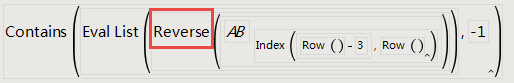Our World Statistics Day conversations have been a great reminder of how much statistics can inform our lives. Do you have an example of how statistics has made a difference in your life? Share your story with the Community!
Choose Language Hide Translation Bar
Highlighted

## How Do I Transfer Excel COUNTIF and MATCH functions into JMP

I am having an issue in scripting the JSL code for two functions (see screen shot) which I currently have in Excel but want to transfer over into JMP. The data is found in the attached file JMP_Test_Average2.xlsx and the functions I wish to transfer to JMP are as follows:

• column VS has a COUNTIF function; =COUNTIF(C3:N3,"-1")
• I want to know the number of instances -1 appears in each row (e.g. in row 3 the number of instances of -1 are 2). Column m(AB) is ignored in the function but it is impoirtant as it is part of the data sequence.
• column PD has a MATCH function; =(MATCH("-1",C3:N3,0))+1
• I want to know where the first instance of -1 appears in each row (e.g. in row 3 the first -1 appears in column m(LM) or column 12 when considering columns m(AB) to m(MN)). Column m(AB) is ignored in the functions but it is impoirtant as it is part of the data sequence.

I would like to know how to script these functions in JSL so I can update my JMP table and not have to complete the calculations in Excel and then bring the results over into my JMP table.

Any help would be much appreciated.

Thanks.

MikeData as found in file JMP_Test_Average2.xlsx

1 ACCEPTED SOLUTION

Accepted Solutions
Highlighted

## Re: How Do I Transfer Excel COUNTIF and MATCH functions into JMP

This should give you a basic Idea.

``````Names Default to here(1);
dt = new table("Test", add rows(10),
New Column("AB", formula(if(random uniform()<=.8, 1, -1))),
New Column("BC", formula(if(random uniform()<=.8, 1, -1))),
New Column("CD", formula(if(random uniform()<=.8, 1, -1))),
New Column("DE", formula(if(random uniform()<=.8, 1, -1))),
New Column("EF", formula(if(random uniform()<=.8, 1, -1))),
);

cols = dt << Get Column Reference(dt << Get Column Names);

dt << new Column("VS", formula(nrows(Loc(Evallist({:AB, :BC, :CD, :DE, :EF}), -1))));
dt << New Column("PD", formula(Contains(Evallist({:AB, :BC, :CD, :DE, :EF}), -1)));``````
Vince Faller - Predictum
12 REPLIES 12
Highlighted

## Re: How Do I Transfer Excel COUNTIF and MATCH functions into JMP

This should give you a basic Idea.

``````Names Default to here(1);
dt = new table("Test", add rows(10),
New Column("AB", formula(if(random uniform()<=.8, 1, -1))),
New Column("BC", formula(if(random uniform()<=.8, 1, -1))),
New Column("CD", formula(if(random uniform()<=.8, 1, -1))),
New Column("DE", formula(if(random uniform()<=.8, 1, -1))),
New Column("EF", formula(if(random uniform()<=.8, 1, -1))),
);

cols = dt << Get Column Reference(dt << Get Column Names);

dt << new Column("VS", formula(nrows(Loc(Evallist({:AB, :BC, :CD, :DE, :EF}), -1))));
dt << New Column("PD", formula(Contains(Evallist({:AB, :BC, :CD, :DE, :EF}), -1)));``````
Vince Faller - Predictum
Highlighted

## Re: How Do I Transfer Excel COUNTIF and MATCH functions into JMP

Thanks for your help, much appreciated.

Highlighted

## Re: How Do I Transfer Excel COUNTIF and MATCH functions into JMP

Here I find the answer and solve my own problems.Thanks!

Contains( Eval List( :BC[Index(Row()-3,Row())] ), -1 )
Highlighted

## Re: How Do I Transfer Excel COUNTIF and MATCH functions into JMP

howdy ! I'm dying to know:
If column "AB" has more than one "-1", how do figure out the number of rows that are within the range of the last "-1" in this range?
Thanks!Highlighted

## Re: How Do I Transfer Excel COUNTIF and MATCH functions into JMP

I tried to write code like this, but it didn't work.Highlighted

## Re: How Do I Transfer Excel COUNTIF and MATCH functions into JMP

This might be the formula you are looking for

``````x = 5 - Contains( Eval List( :ab[Index( Row(), Row() - 3 )] ), -1 );
If( x == 5, x = 0 );
``````
Jim
Highlighted

## Re: How Do I Transfer Excel COUNTIF and MATCH functions into JMP

Thank Jim!

x=5-Contains(Eval List(:ab[Index(Row(),Row()-3)]),-1);
If(x==5,0,x);
Highlighted

## Re: How Do I Transfer Excel COUNTIF and MATCH functions into JMP

``````//this gives you a vector of items that are -1
vector_of_neg1 = loc(Aslist(:AB[row()-3::row()]`), -1);
//then you just have to select the last one
n = nitems(vector_of_neg1);
if(n,
vector_of_neg1[n]
, //else 0
0
);
``````

Or you can use the start argument to look from the back.

``Contains(  :AB[Index( Row() - 3, Row() )] , -1, -1 )``

Vince Faller - Predictum
Highlighted

## Re: How Do I Transfer Excel COUNTIF and MATCH functions into JMP

How can the column name table {:AB, :BC, :CD, :DE, :EF} of each column in the code be automatically generated by the code?

{:AB, :BC, :CD, :DE, :EF}

Thanks!

I've tried the following code to have the same effect, but it still doesn't generate automatically.

Evallist({As Column(1),As Column(2),As Column(3),As Column(4),As Column(5)})

Article Labels

There are no labels assigned to this post.